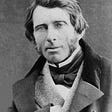# What’s Atomic Packing Factor?

Atomic packing factor (APF) is the ration of volume of the number of atoms that contribute to unit cell, to the whole volume of the unit cell. It is the fraction of volume in a crystal structure that is occupied by constituent particles. Atomic packing factor tells you what percent of an object is made up of atoms versus empty spaces. It is also known as packing fraction or packing efficiency. Atomic packing factor is a dimensionless quantity. Its value is always less than unity.

The formula of APF :

APF= Number of atoms per unit cell x Volume of each atom / Volume of each unit cell

Lets understand more about it by solving its value for some types of unit cells.

For Primitive Cubic:

In primitive cubic or simple cubic unit cell there is one atom present at each corner. Since, there are eight corners so, the contribution of each atom is 1/8 because each atom is shared by total eight unit cells present in the surrounding.

Number of atoms per unit cell = 8x1/8 =1

The volume of single atom= 4/3 πr³ , it’s the volume of sphere as the atom shape.

The volume of unit cell =height x length x width

Lets consider one side equals to a

So, the volume of the unit cell = a x a x a = a3

As a = 2r for primitive cubic unit cell

So, APF = 1 x (4/3 πr³) / (2r)3 = π/6 = 0.52 0r 52%

Thus, the APF value for simple cubic unit cell is 52%. That means 52% portion of the simple cubic unit cell is covered and 48% is an empty space.

For Body Centered Cubic:

In body centered cubic unit cell there is one atom at each corner and one additional is present in the center, as shown above, of the unit cell.

The contribution of each corner atom is 1/8.

So, Number of atoms =8 x 1/8 = 1

No. of central atom = 1

Total number of atoms = 1+1 = 2

The volume of single atom= 4/3 πr³ , it’s the volume of sphere as the atom shape.

The volume of unit cell =height x length x width

Lets consider one side equals to a

So, the volume of the unit cell = a x a x a = a3

APF = 2 x (4/3 πr³) / a3

For BCC, a= 4r/ √3

So, APF =2 x (4/3 πr³) / (4r/ √3)3

APF = π √3 / 8 =0.68 or 68%

Thus, the APF value for body centered cubic unit cell is 68%.

For Face Centered Cubic:

In face centered cubic unit cell there is one atom at each corner and total of six atoms present at faces, as shown above, of the unit cell.

The contribution of each corner atom is 1/8.

So, number of atoms =8 x 1/8 = 1

The contribution of face atoms= ½

As there are 6 faces so, total number of face atoms = 6 x ½ = 3

Total no. of atoms= 3 + 1 =4

The volume of single atom= 4/3 πr³ , it’s the volume of sphere as the atom shape.

The volume of unit cell =height x length x width

Lets consider one side equals to a

So, the volume of the unit cell = a x a x a = a3

APF = 4 x (4/3 πr³) / a3

For FCC the value of a = 4r/ √2

So, APF = 4 x (4/3 πr³) / (4r/ √2)3

APF= π √2 / 6 =0.74 or 74%

Thus, the APF value for face centered cubic unit cell 74%.

Atomic packing factor influences the type of material such as a metal having high value of APF is more malleable or ductile because unit cells are closer in this type of structure allows metal atoms to slide on one another easily.

For further understanding watch this video: https://www.youtube.com/watch?v=rroulXtAxXk

--

--

--

## More from S Saleha

Welcome everyone! I am a wander writer who writes about everything.

Love podcasts or audiobooks? Learn on the go with our new app.

## The Tale of Pythagorean Formula## Time? of course there’s an instance of Time in the Universe! it’s not your classic version tho…## Part 1: Matrix Definitions## Vulcan Forge will melt faces due to insane yield potential.## NCRT Question chapter motion class 9## S Saleha

Welcome everyone! I am a wander writer who writes about everything.

## Post-truth Filipino Society: Where Lies Triumph Over Facts## John Ruskin — An Analysis of The Storm Cloud of the Nineteenth Century and Conservative Scholars